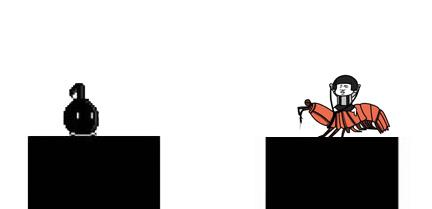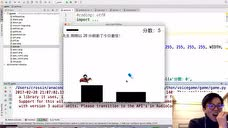魔性声控游戏 - 暴走皮皮虾 - 腾讯视频https://v.qq.com/x/page/z0380ogn3e7.html

## pyaudio

• 定义角色，添加在场景上
``````# 定义 sprite
class PPX(cocos.sprite.Sprite):
def __init__(self):
super(PPX, self).__init__('ppx.png')
... # 略
# 创建并添加
self.ppx = PPX()
• 给角色增加重力的效果
``````def update(self, dt):
self.speed += 10 * dt
self.y -= self.speed
if self.y < -80:
self.reset()``````
• 获取麦克风的声音
``````# 初始化
pa = PyAudio()
SAMPLING_RATE = int(pa.get_device_info_by_index(0)['defaultSampleRate'])
self.stream = pa.open(format=paInt16, channels=1, rate=SAMPLING_RATE, input=True, frames_per_buffer=self.NUM_SAMPLES)
# 每帧中获取音量
k = max(struct.unpack('1000h', string_audio_data))``````
• 通过音量判断行走和跳跃
``````if k > 3000:
self.floor.x -= min((k / 20.0), 150) * dt
if k > 8000:
self.ppx.jump((k - 8000) / 1000.0)
``````

• 简单的碰撞检测
``````def collide(self):
px = self.ppx.x - self.floor.x
for b in self.floor.get_children():
if b.x <= px + self.ppx.width * 0.8 and px + self.ppx.width * 0.2 <= b.x + b.width:
if self.ppx.y < b.height:
self.ppx.land(b.height)
break``````